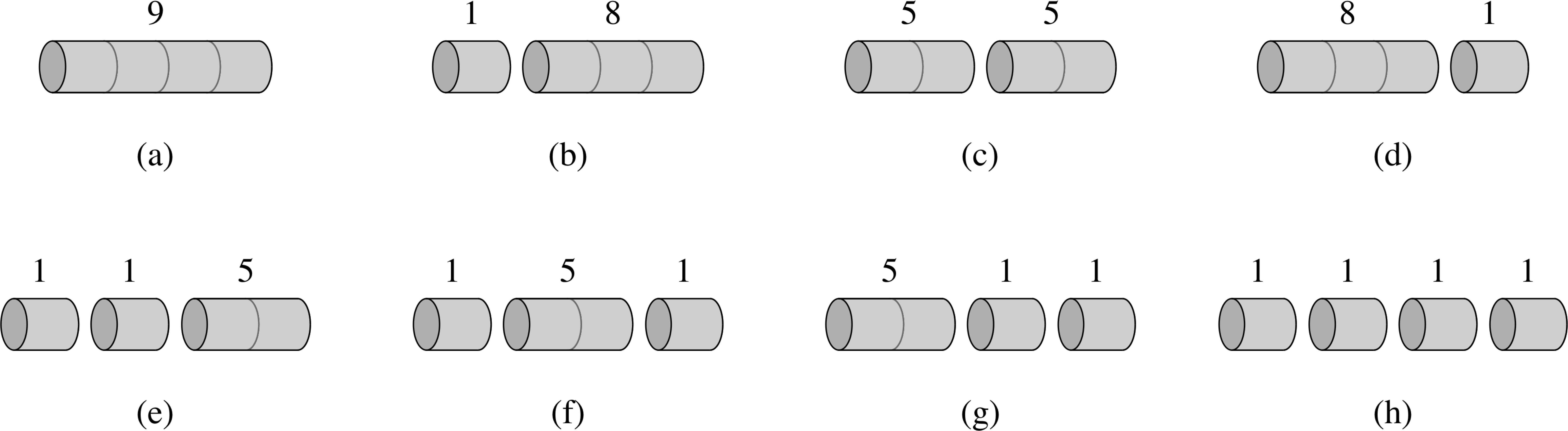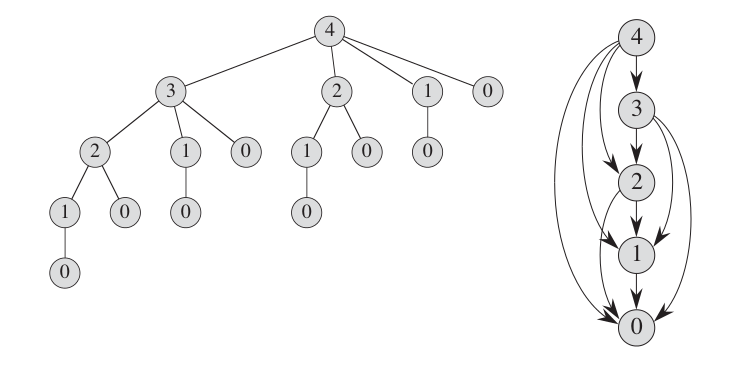×

Search anything:

# Rod cutting problem

#### Algorithms Dynamic Programming (DP)Get this book -> Problems on Array: For Interviews and Competitive Programming

Reading time: 25 minutes | Coding time: 5 minutes

The problem statement is quite simple, given a rod of some length 'n' and the price associated with each piece of the rod, the rod has to be cut and sold.The process of cutting should be in such a way that the amount(revenue) obtained from selling is maximum. Also given, the amount is different for different sizes.

By the end, you should be able to answer this deep question: If there are 2^(N-1) possibilities, how are we able to solve it in O(N^2)? This is focused on how we are handling possibilities through Dynamic Programming.

Whenever we see the keyword "minimum", "maximum" these problems can be categorized under optimization problems, due to it's nature of finding an optimal value to perform a task. Some examples of these kind of problems include knapsack problem, longest common subsequence, etc. There are many ways to solve this kind of problems, however, dynamic programming works the best. Let's check out the brute force approach following the detailed explanation of the problem statement.

length price
1 1
2 5
3 8
4 9
5 10
6 17
7 17
8 20
9 24
10 30Given a sample price table for rods. Each rod of i inches can generate price i revenue.

The above figure depicts 8 possible ways of cutting up rod of length 4. Above each piece is given the price of that piece according to the table. The optimal way of cutting the rod is c since it gives maximum revenue(10).

We have taken two approaches to solve this problem:

• Brute Force approach O(2^(N-1)) time
• Dynamic Programming approach O(N^2) time

# Brute Force approach

For a rod of length n, since we make n-1 cuts, there are 2^(n-1) ways to cut the rod. Also for every length, we can either choose to cut the rod or not.

The basic idea is that given a length N, the maximum profit is the maximum of the following:

``````price[i] + max_price(N - i)
``````

which means:

The rod of length N is being split into a part of length i which has price as price[i] and the rest of the rod of length N-i is split further which is captured by the recursive function max_price(N-i).

You need to check for all possible values of i.

The given code is a recursive C++ approach for the given logic.

``````#include<bits/stdc++.h>
using namespace std;
int rodCutting(int price[], int len)
{
if(len<=0)
return 0;
int max_len=INT_MIN;
for(int i=0; i<len; i++)
{
max_len = max(max_len, price[i] + rodCutting(price, len-(i+1)));
}
return max_len;
}

int main(){
int price = {1,5,8,9,10,17,17,20,24,30};
int rod_len=4;
cout<<rodCutting(price,rod_len,10)<<'\n";
}
``````

Output:

``````10
``````

Time Complexity: O(2^(N - 1))

We get this exponential complexity due to repeatedly calling the same methods again and again.

What the algorithm does?

The variable max_len is initialized to minimum value possible. Then, we run a loop until the given rod length (in this case 4), to find the maximum value between the previous max_len and the value obtained by adding the current price and result of recursively calling the function for len-i+1 value.

For rod length 4, there are 2^(3) i.e 8 ways of cutting it, we can cut it in (3+1), (2+2), (1+1+1+1)....ways.

So the algorithm calculates in a top down approach the maximum revenue for rod length 1,2,3 to get the final answer. The recursion tree would explain it more clearly.The recursion tree shows a recursive call resulting from rodCutting(price,4). This figure clearly explains why the computation time for the algorithm is so ridiculous. We keep calling the function again and again even though the result has already been calculated. For example rodCutting(1) has been calculated 4 times.In order to avoid that we use dynamic programming.

# Dynamic Programming approach

To understand why need can use Dynamic Programming to improve over our previous appraoch, go through the following two fundamental points:

1. Optimal substructure

To solve a optimization problem using dynamic programming, we must first characterize the structure of an optimal solution. Specifically, we must prove that we can create an optimal solution to a problem using optimal solutions to subproblems. We can’t really use dynamic programming if the optimal solution to a problem might not require subproblem solutions to be optimal. This often happens when the subproblems are not independent of each other.

For cutting the rod of length 4, we considered cutting it into 2,2 would be optimal. Even for the 2 we choose, there are optimal ways to cut that as well. (We can store all these intermediate results)

1. Overlapping subproblems

For dynamic programming to be useful, the recursive algorithm should require us to compute optimal solutions to the same subproblems over and over again, because in this case, we benefit from just computing them once and then using the results later. In total, there should be a small number of distinct subproblems (i.e. polynomial in the input size), even if there is an exponential number of total subproblems.

We'll be using a table to store all the intermediate results, the core logic will remain same, but the way it way it will be implemented will change.

The idea is same as the previous approach with the only difference that the values of max_price(N-i) in:

``````price[i] + max_price(N - i)
``````

are precomputed and stored in an array. This removes all duplicate calls and optimizes our solution greatly.

Go through this code to understand this approach better (we have explained the working following the implementation as well):

``````#include<bits/stdc++.h>
using namespace std;
int rodCutting(int price[], int len){
int val[len+1];
val=0;
int i,j;

// Build the table val[] in bottom up manner and return the last entry
// form the table
for (i = 1; i<=len; i++)
{
int max_val = INT_MIN;
for (j = 0; j < i; j++)
max_val = max(max_val, price[j] + val[i-j-1]);
val[i] = max_val;
}

return val[n];
}

int main(){
int price = {1,5,8,9,10,17,17,20,24,30};
int rod_len=4;
cout<<rodCutting(price,rod_len,10)<<'\n";
}
``````

Output:

``````10
``````

For rodCutting(4) the memoization table is as follows

val
0
1
5
8
10

For filling this table we run a nested loop to calculate val[i], where i represents the length of the rod, and the val[i] contains the optimal revenue that would be obtained if the rod were to be cut in i pieces.
This table solves the overlapping sub-problems part very quick, as we already save the intermediate results, we don't have to re-calculate it.

`````` for (i = 1; i<=len; i++)
{
int max_val = INT_MIN;
for (j = 0; j < i; j++) {
max_val = max(max_val, price[j] + val[i-j-1]);
val[i] = max_val;
}
}
``````

Just like the brute force approach we take a max_val that is initialized to a minimum value. We build the solution in a bottom up approach here.

``````max_val = max(max_val, price[j] + val[i-j-1])
``````
• The price[j] + val[i-j-1] simply calculates the current price plus the previous(already calculated optimum value)value from the val[] array. The max_val calculation is done by comparing the maximum value between previous max_val and the current price[j] + val[i-j-1].
• For val we run the j loop from 0 to 1, counting in all the 2^(1-1) possibilities.
• For val we run the j loop from 0 to 2, counting in all 2^(2-1) possibilities i.e. (1+1), (2). since we already computed val we won't be doing it again.
• For val we build the solution in the same way by considering 2^(3-1) approaches(1+2),(1+1+1),(2+1),(3). Unlike the previous method, since we already have those values (val, val) we won't be calculating again.
• For val we chose among (1+1+1+1), (1+3), (2+2), (3+1), (1+1+2), (1+2+1), (2+1+1), (4).

We are starting the loop from 1 as that's our starting point.

The values and their respective implementation of our memoization table i.e val[] is given as follows

• val=0 //a hypothetical situation, there isn't really a rod of length 0
• val=1 //maximum revenue if the rod is of length 1, there isn't much calculation as there is only one answer for a rod length of 1
• val=5//we need to find max of the total 2^(1) possibilities, the only difference here is instead of recalculating we use the values that are already existing.
• val=8 // calculated in the same way by taking in all the 4 possibilities and returning 8
• val=10 // calculation is done in the same way, as the previous

Time Complexity: O(n^2)

This time complexity is till better than the worse time complexity of the brute force approach.

# Applications

1. The approach explained here can be applicable to many dynamic programming questions directly like the fibonacci series, and indirectly be used to understand other questions like coin change problem, longest common subsequence(LCS) etc.
2. The dynamic programming approach is very useful when it comes to optimization problems like the graph algorithms(All pair shortest path algorithm) that are extensively applied in real-life systems.
3. The others include 0/1 knapsack problem, Mathematical optimization problem, Reliability design problem, Flight control and robotics control, Time sharing: It schedules the job to maximize CPU usage.

Differences between brute force and dynamic programming approaches for this problem

1. The complexity in the case of dynamic programming has significantly improved from exponential to n^2.
2. Brute force is a recursive top down, where as dynamic programming is a bottom up construction for the memoisation table.
3. Using this table has helped us from redundant calculations in the case of brute force.
4. Although using a table would impose additional space complexity (O(n)), but since this improves the running time of the algorithm, this works.

#### How many ways are there to cut a rod of length n?

2^(n-1)
2^n
2^n -1
n^2
For a rod of length n, since we make n-1 cuts, there are 2^(n-1) ways to cut the rod.

Ask yourself: If there are 2^(N-1) possibilities, how are we able to solve it in O(N^2)? This is focused on how we are handling possibilities through Dynamic Programming.

With this, you will have the complete knowledge of solving the rod cutting problem. Enjoy.#### K. Sai Drishya

Love music; Student at Gandhi Institute of Technology and Management (GITAM); Intern at OpenGenus